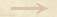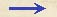Other Propositions Aristot-space-prop Other PropositionAristot-space-propThe Aristotelian Proportion between Velocity and Space In Ms. Gal. 72 Galileo made use of two relations between velocities, distances and times which were common in the Aristotilian tradition. He later integrated these relations as propositions into the deductive theorie of motion presented in De motu locali. One of these is there formulated as 1/02-th-02: If a moving particle traverses two distances in equal intervals of time, these distances will bear to each other the same ratio as the speeds. And conversely if the distances are as the speeds then the times are equal. (See also Aristotle, Physics, 232a23-232b15.)Other Propositions Aristot-space-prop Other PropositionAristot-space-prop